# How to Add Text at the Beginning or End of All Cells in Excel?

Let's suppose you need to add a particular text string in all the cells of an Excel sheet at the beginning or end of the existing data. It can be a tedious process if you decide to perform this such a task manually. In this tutorial, we will show you a simple method by which you can do it using a formula in Excel.

We can add text in beginning or end using the same formula but the process is sequence of the work is different. Let us see the simple process to add text in bigging or end of all cells

## How to Add Text to the Beginning of All Cells in Excel?

We will essentially use the same formula to add text at the beginning or end of selected cells, but the process is slightly different for both.

For adding text at the beginning of a cell, we will use the formula −

= "data" & address of data

For adding text at the end of a cell, we will use the formula −

= address of data & "data"

Let's take an example and see how it is implemented.

### Step 1

Open an Excel sheet and insert some dummy data in a column, as shown below −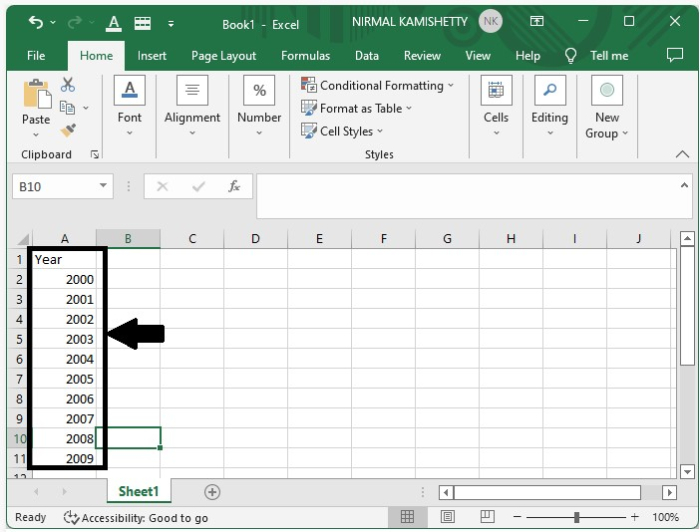### Step 2

Select a cell where you want to display the first result and then type the formula ="data"&A2 in the formula box. Here, we are adding the text "data" at the beginning of the cell.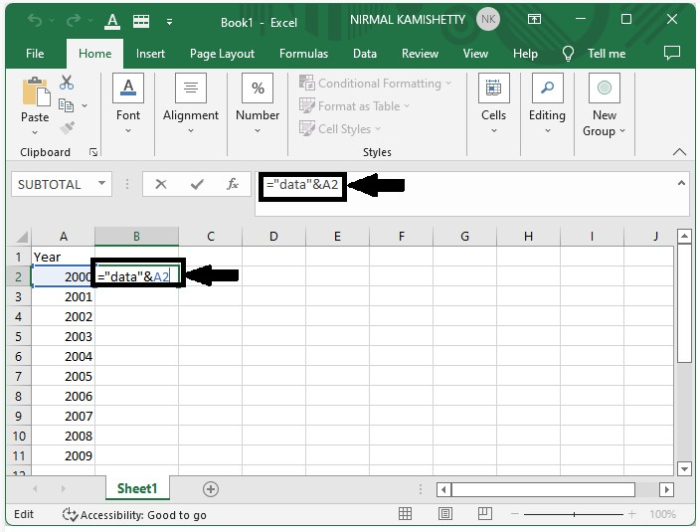Press the "Enter" button to get the first result.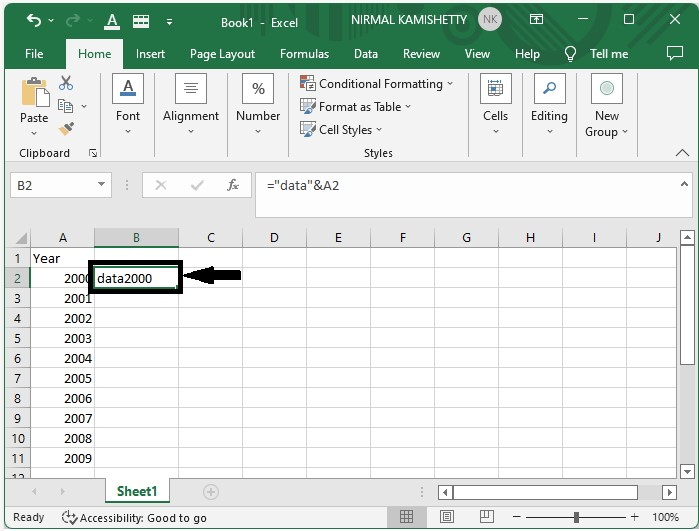### Step 3

You have successfully updated the first item. Similarly, you can update all the data values just by dragging down from the right corner of the cell where the first result is displayed. The final screen will appear like the one shown below −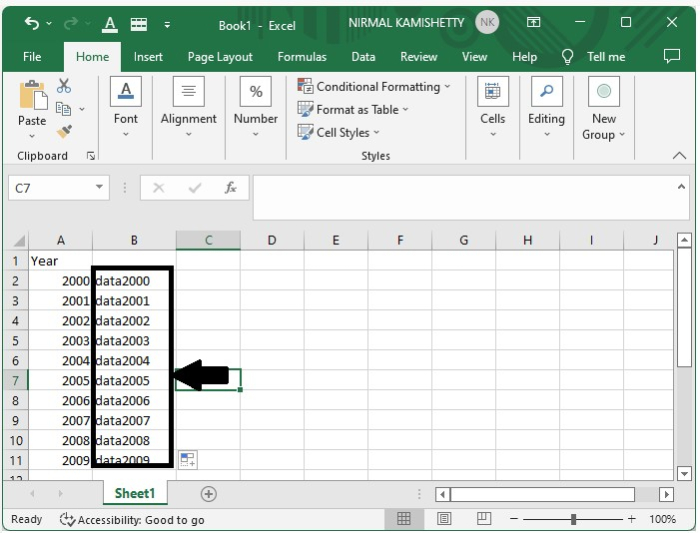## How to Add Text at the End of All Cells in Excel?

We successfully added data before the text now let us the process to add the data after provided data

### Step 1

For adding the text after the number let us consider the same data which we used in the above example

type the formula as =A2&"data" in the formula box after clicking on the cell where we want to create our result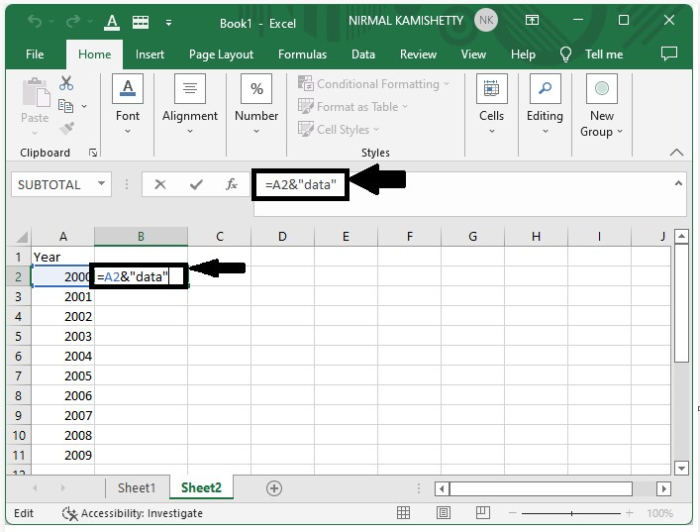Click enter to get our first result as shown in the below figure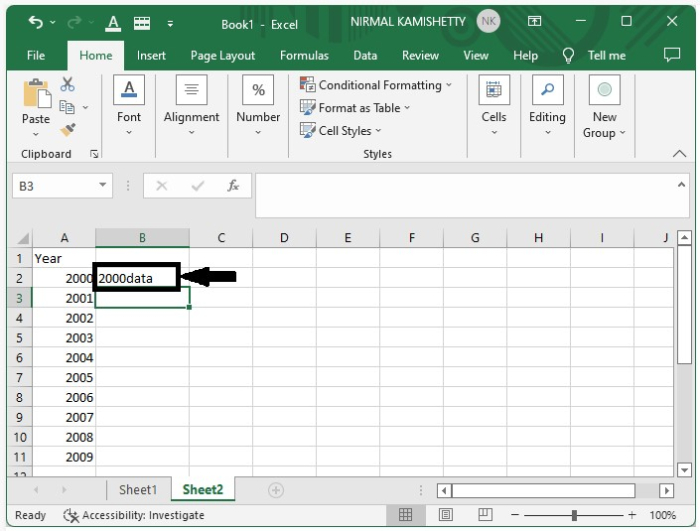### Step 2

We have successfully created the result for the first item we create results for all the remaining data just by dragging down from the right corner of the cell where the result is already present and our final result will look like the below image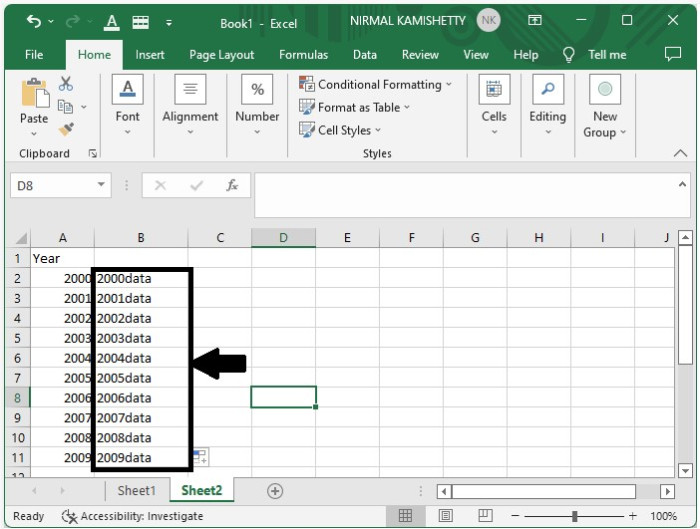This is how we can add text at end of the data for all the cells in excel

Tip − In the formula, the data that is to be added must be present inside the double quotes

("").

## Conclusion

In this tutorial, we used a simple example to demonstrate how you can add text at the beginning or end of all cells in Excel.